### Home > PC > Chapter 4 > Lesson 4.3.1 > Problem4-128

4-128.
1. Find the exact value of each of the following trig expressions. Homework Help ✎

1. sin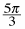2. cos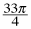3. cot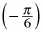4. sec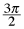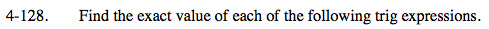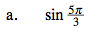1. Plot the point on a unit circle.
2. Find the sides of this special triangle.
3. Be careful of the signs!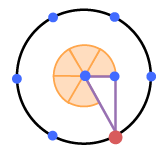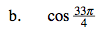$\frac{32\pi}{4} \text{ bring the point back to }(1,0).$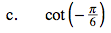Wrap clockwise because of the negative sign.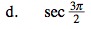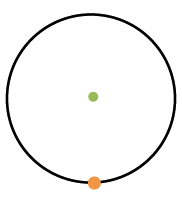$\text{Since the sec\theta; is }\frac{1}{\text{cos}\theta}, \text{ this would be undefined}$

$\text{because the cos=0 at }\frac{3\pi}{2}.$